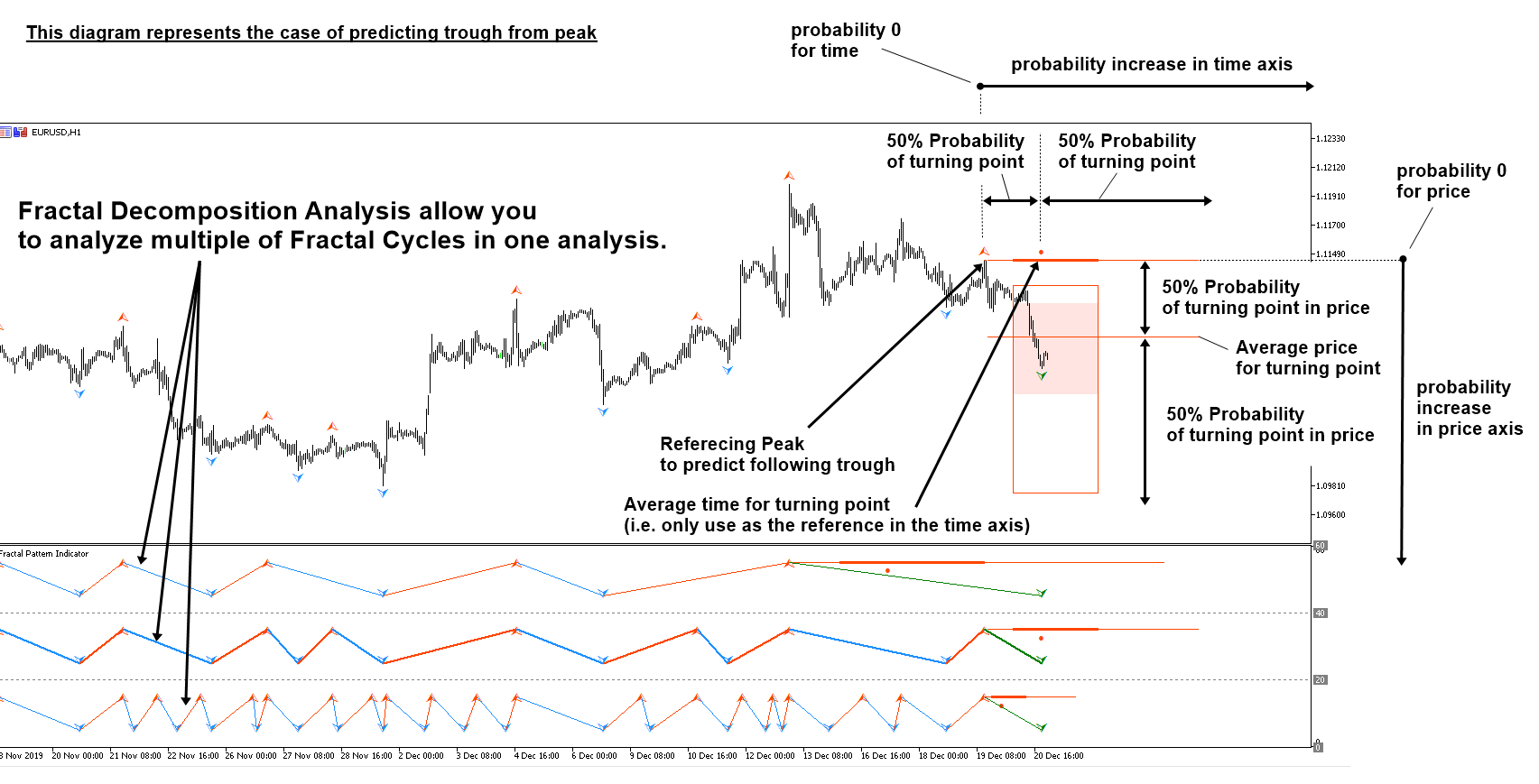# Average Price and Time of Turning Point in the Financial Market

Fractal Decomposition Analysis (FDA) is the first technique, originally developed by Young Ho Seo, that allow us to view the turning point in the financial market through the detailed probability analysis. since FDA allow us to use multiple of Fractal Cycle analysis, you can also called this technique as Fractal Cycle Analysis (FCA).

Here we will share some of its anatomy involved in predicting Turning point using probability using FDA. In the screenshot, we shows the average price and time of turning point. Average price of turning point is where it divides the price axis into two section with equal 50% probability. Likewise, average time of turning point is where it divides the time axis into two section with equal 50% probability.

Also average price can be considered as the expected price range before turning point occurs. Likewise, average time can be considered as the expected time range before turning point occurs. When we say expected values in statistics, it means that actual turning point occurrences can be fluctuating around these expected values (=mean).

As you can see from the screenshot, average price and time is not in the middle of the distribution. You wonder why this is the case. The distribution, empirical distribution measured, is not symmetric. Distribution is skewed. Hence, we have thin and long right tail.

Theoretically speaking, small Fractal cycle would be slightly more accurate. However, medium and large cycle are both good reference for our trading and predicting next turning point. We recommend to overview all these three cycles when you make the trading decision.

As a default setting, we have inner probability and outer probability as 68% and 95% respectively. These two probability setting can be used to filter out some of the probability you do not want to use. At the same time, you can change the inner probability and outer probability as any values you want as long as inner probability is smaller than outer probability. You might consider using 80% and 90% values for the setting.

Here is the landing page for Fractal Pattern Scanner for MetaTrader 4 and MetaTrader 5.

https://www.mql5.com/en/market/product/49170

https://www.mql5.com/en/market/product/49169